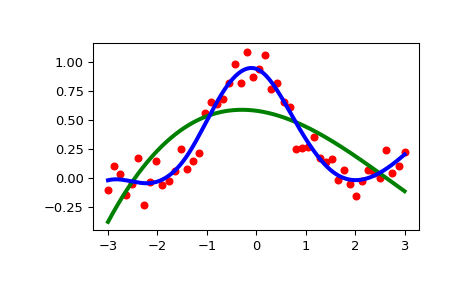# scipy.interpolate.UnivariateSpline¶

class scipy.interpolate.UnivariateSpline(x, y, w=None, bbox=[None, None], k=3, s=None, ext=0, check_finite=False)[source]

1-D smoothing spline fit to a given set of data points.

Fits a spline y = spl(x) of degree k to the provided x, y data. s specifies the number of knots by specifying a smoothing condition.

Parameters
x(N,) array_like

1-D array of independent input data. Must be increasing; must be strictly increasing if s is 0.

y(N,) array_like

1-D array of dependent input data, of the same length as x.

w(N,) array_like, optional

Weights for spline fitting. Must be positive. If None (default), weights are all equal.

bbox(2,) array_like, optional

2-sequence specifying the boundary of the approximation interval. If None (default), bbox=[x, x[-1]].

kint, optional

Degree of the smoothing spline. Must be 1 <= k <= 5. Default is k = 3, a cubic spline.

sfloat or None, optional

Positive smoothing factor used to choose the number of knots. Number of knots will be increased until the smoothing condition is satisfied:

sum((w[i] * (y[i]-spl(x[i])))**2, axis=0) <= s


If None (default), s = len(w) which should be a good value if 1/w[i] is an estimate of the standard deviation of y[i]. If 0, spline will interpolate through all data points.

extint or str, optional

Controls the extrapolation mode for elements not in the interval defined by the knot sequence.

• if ext=0 or ‘extrapolate’, return the extrapolated value.

• if ext=1 or ‘zeros’, return 0

• if ext=2 or ‘raise’, raise a ValueError

• if ext=3 of ‘const’, return the boundary value.

The default value is 0.

check_finitebool, optional

Whether to check that the input arrays contain only finite numbers. Disabling may give a performance gain, but may result in problems (crashes, non-termination or non-sensical results) if the inputs do contain infinities or NaNs. Default is False.

InterpolatedUnivariateSpline

Subclass with smoothing forced to 0

LSQUnivariateSpline

Subclass in which knots are user-selected instead of being set by smoothing condition

splrep

An older, non object-oriented wrapping of FITPACK

splev, sproot, splint, spalde
BivariateSpline

A similar class for two-dimensional spline interpolation

Notes

The number of data points must be larger than the spline degree k.

NaN handling: If the input arrays contain nan values, the result is not useful, since the underlying spline fitting routines cannot deal with nan. A workaround is to use zero weights for not-a-number data points:

>>> from scipy.interpolate import UnivariateSpline
>>> x, y = np.array([1, 2, 3, 4]), np.array([1, np.nan, 3, 4])
>>> w = np.isnan(y)
>>> y[w] = 0.
>>> spl = UnivariateSpline(x, y, w=~w)


Notice the need to replace a nan by a numerical value (precise value does not matter as long as the corresponding weight is zero.)

Examples

>>> import matplotlib.pyplot as plt
>>> from scipy.interpolate import UnivariateSpline
>>> x = np.linspace(-3, 3, 50)
>>> y = np.exp(-x**2) + 0.1 * np.random.randn(50)
>>> plt.plot(x, y, 'ro', ms=5)


Use the default value for the smoothing parameter:

>>> spl = UnivariateSpline(x, y)
>>> xs = np.linspace(-3, 3, 1000)
>>> plt.plot(xs, spl(xs), 'g', lw=3)


Manually change the amount of smoothing:

>>> spl.set_smoothing_factor(0.5)
>>> plt.plot(xs, spl(xs), 'b', lw=3)
>>> plt.show()Methods

 __call__(self, x[, nu, ext]) Evaluate spline (or its nu-th derivative) at positions x. antiderivative(self[, n]) Construct a new spline representing the antiderivative of this spline. derivative(self[, n]) Construct a new spline representing the derivative of this spline. derivatives(self, x) Return all derivatives of the spline at the point x. get_coeffs(self) Return spline coefficients. get_knots(self) Return positions of interior knots of the spline. get_residual(self) Return weighted sum of squared residuals of the spline approximation. integral(self, a, b) Return definite integral of the spline between two given points. roots(self) Return the zeros of the spline. set_smoothing_factor(self, s) Continue spline computation with the given smoothing factor s and with the knots found at the last call.
 validate_input

#### Previous topic

scipy.interpolate.insert

#### Next topic

scipy.interpolate.UnivariateSpline.__call__